Preeti Rai

student | Physics Chemistry Biology Maths English Indian Economy Development

Description

Hi I am student of class 12, science student from CBSE board.

Probabilities of mutually exclusive, exhaustive and equally likely events

Probabilities of mutually exclusive, exhaustive and equally likely events

Let E1, E2, ..., En be n mutually exclusive, exhaustive and equally likely events. Then P(E1 ∪E2....∪En)=1 (since exhaustive events)
But P(E1 ∪E2....∪En)=P(E1)+P(E2)+ .....+P(En)(since mutually exclusive events) ⇒ P(E1)+P(E2)+....+P(En)=1
Also P(E1) = P(E2) = ..... = P(En) (Since equally likely events)

nP(Ei)=1,i∈{1,2,3,.....n} ⇒ P(Ei)=1/n

So P(E1) = P(E2) = .... = P(En) = 1/n

So probability of each of n mutually exclusive, exhaustive and equally likely events is 1/n .

How to remember periodic table easily || best tricks in Hindi

How to remember periodic table easily || best tricks in Hindi

Density of Crystal - Solid State

Density of Crystal

If length of edge of unit cell  = l

Volume of unit cell =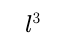Density of unit cell =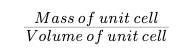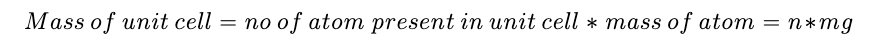Mass of one atom (m). =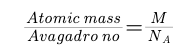Mass of unit cell  =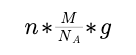Density of unit cell =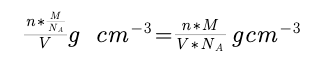Question :

Gold Au(197) crystellises in F.C.C whose crystal density is 19.3 gm/cm^3 .Calculate atomic radius of gold atom.

Solution :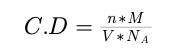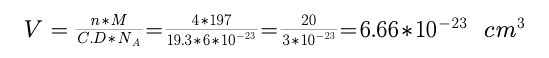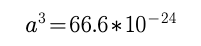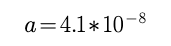In F.C.C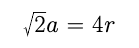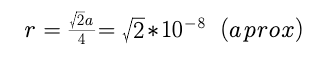Maths important formula - BINOMIAL THEOREM

BINOMIAL THEOREM

1.Statement of Binomial Theorem:

if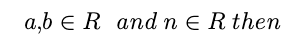2. Properties of Binomial Theorem:

i. General term :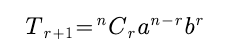ii. middle term(s):

a. if n is even, there is only one middle term which is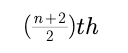term.

b. if n is odd, there are two middle terms which are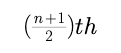and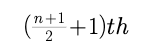term

3.Multinomial Theorem :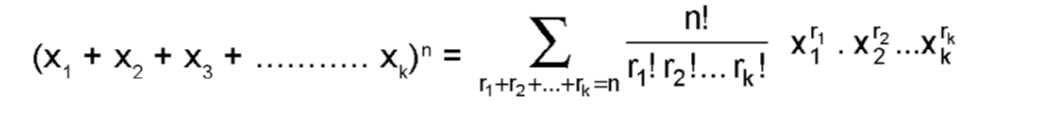Here total number of terms in the expansions =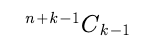4. Application of Binomial Theorem:

if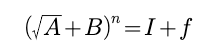where I and n are positive intergers, n beign odd and 0 < f <1 then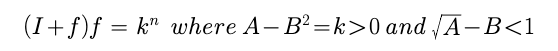if n is an even integer, then (I+f)(I - f )= k^n

5. Properties of Binomial Coefficients :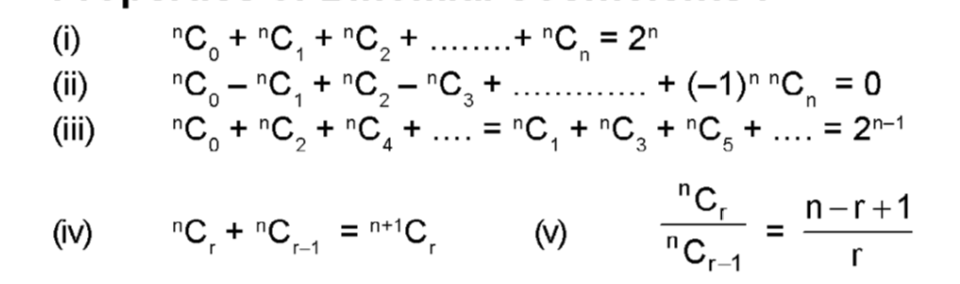Thermodynamics - First and second law of thermodynamics; Internal energy; enthalpy, work and heat

Thermodynamics

The study of the transformation of energy is called thermodynamics. Actually, it deals with energy in its various forms, which include thermal, chemical, electrical, and mechanical, with the restrictions on the transformation of one type of energy in to the other types and with the relation of energy changes to physical and chemical changes.

Terminology of Thermodynamics

System and Surroundings

System is part of the universe which is arbitrarily set off from the rest of the universe by definite boundaries for the purpose of experimental or theoretical studies. The remainder of the universe is then, in fact the surroundings of the system. The space separating the system from its surrounding is termed as boundary.

Types of Systems

a)  Real system -    In experimental work, the system is called Real.

b)  Ideal system -In pencil and paper work, the system treated is called ideal. An ideal system is always considered to simplify the thermodynamic problems.

c)  Isolated system - A system is said to be isolated when it can neither exchange energy nor matter with its surroundings.

d)  Closed system. - A system is said to be closed when it permits passage of energy but not mass, across the boundary.

e)  Open system - A system which can exchange both energy and matter with its surroundings.

State of System (State variables)

The quantities whose value serve to describe the system completely are called the thermodynamic properties of the system. Once the properties of the system are completely specified, one says that the state of the system is specified. Thus, the defining properties are sometimes called state variables or state properties. Examples of state properties are pressure, volume, temperature and composition. The question now arises as how many variables must be determined to define the system completely. The answer to this question can be obtained by considering the following example.

A homogeneous system consists of a single substance and hence the composition is fixed automatically. The state of a homogeneous system can, therefore, be defined by only three variables:

i)  Pressure

ii)  Volume and

iii)  Temperature

For a homogenous system of definite mass, these three properties are related to one another by a mathematical equation PV = RT, called equation of state. With the help of this equation (PV = RT) the values of any one of these properties can be determined knowing the values of the other two properties. Therefore, state of a simple homogeneous system may be completely defined by specifying only two of the three variables (i.e.) pressure, temperature and volume. The two variables generally specified are temperature and pressure. These are termed as independent variables. The third variable, generally volume is called a dependent variable because its value depends upon the values of pressure and temperature.

When we are considering a closed system consisting of one or more components, mass is not a state variable.

In order to define the state of a homogeneous system having more than one substance, one must consider and describe each of the phases of the system. For each phase, one must specify the content (i.e.) the amount of each substance present, and two other independent variables.

In order to define a system completely, the state variables are generally (T), Pressure (P) Volume (V) and concentration (n). Besides these there are two more variables. Work (W) and heat (q), which are not state properties. These six variables play an important role in defining chemical systems completely.

Properties of a System: The observable properties of system are of two types:

a)  Extensive Properties: There are some properties called Extensive properties whose values are proportional to the mass of the portion of the system or one can say that extensive properties are dependent upon size of the system.

b)  Intensive Properties: There are some properties of a system called intensive properties, whose values are independent of the quantity of matter contained in the system.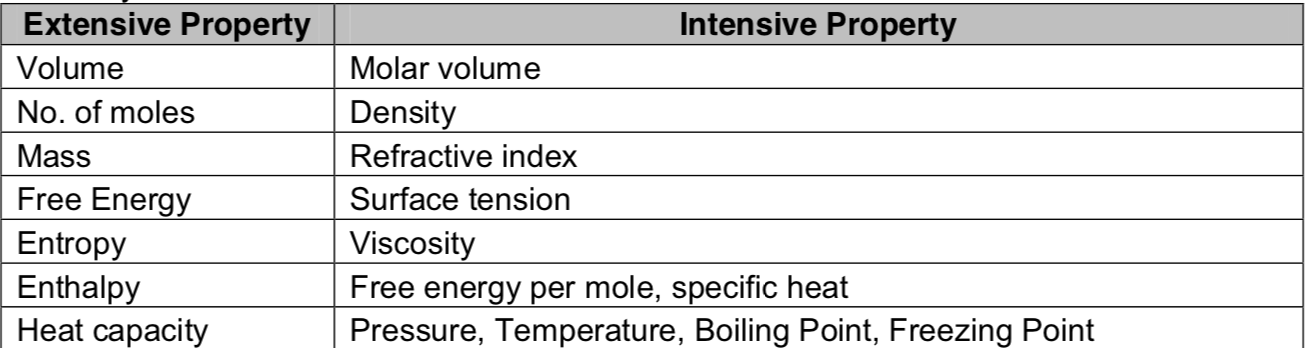Internal Energy

It is the energy associated with a system by virtue of its molecular constitution and the motion of its molecules. The contribution of energy due to molecular constitution is known as cinternal potential energy and the contribution of energy due to the motion of molecules is called internal kinetic energy. Internal energy of a system is given by the sum of two types of energies.

Determination of E: When a reaction is carried out in such a manner that the temperature and volume of the reacting system remain constant, then the internal energy change (E) of the reaction is equal to the heat exchanged with the surrounding.

Thermodynamic Process

(i)Adiabatic Process: When a process is carried out under such conditions that no exchange of heat takes place between the system and its surrounding, the process is called adiabatic.

Ex: The sudden bursting of a cycle tube. If the process is exothermic the heat evolved will remain in the system and, therefore, the temperature of the system rises and vice versa.

ii) Isothermal Process: The process which occurs at constant temperature is called isothermal. In an isothermal change the temperature is kept constant by adding heat or taking it away from the substance. So in an isothermal process the system exchanges heat with the surroundings.

iii) Isobaric Process: If the pressure of the system remains constant during each step of the change in the state of a system, this process is said to be an isobaric process.

iv) Isochoric process: A process is defined to be isochoric if the volume of the system remains constant during the process.

First law of Thermodynamics

Energy may be converted from one form to another, but it is impossible to create or destroy it. There are various ways of expressing the first law of thermodynamics. Some of the selected statements are given below:

i)  When work is transformed into heat or heat into work, the quantity of work is mechanically equivalent to the quantity of heat.

ii)  Energy of an isolated system must remain constant, although it may be transformed from one form to another.

iii)  Energy in one form, if it disappears will make its appearance in an exactly equivalent in another form.

iv)  It is never possible to construct a perceptual motion machine that could produce work without consuming any energy.

Mathematical Formulation of the First Law

Suppose a system absorbs a quantity of heat q and its state change form A to B (fig – I). This heat is used up,

i) In increasing the internal energy of the system i.e,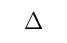E = EB – EA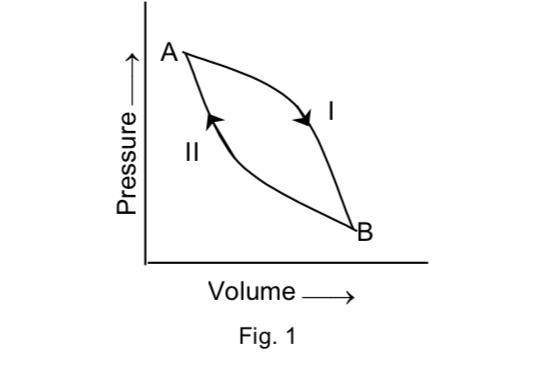ii) To do some external work ‘w’ by the system on its surroundings.
From the first law, we get.
Heat observed by the system = its internal energy + work done by the system.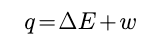Differential form of the First Law

For an infinitesimal process, equation (1) takes the form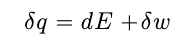a).Change in internal energy is independent of the path taken. So in mathematical terms, an exact differential is always denoted by a notation d, e.g. dE in equation (2).

b)  q and w are not state functions because changes in their magnitude is dependent on the path by which the change is accomplished. Mathematically q & w are not exact differentials and we always write the inexact – differential by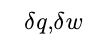etc.

c)  For a cyclic process, the change in the internal energy of the system is zero because the system is brought back to the original condition.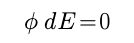or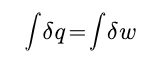i.e

the total work obtained is equal to the net heat supplied.

The sign convention: According to latest S.I. convention, w is taken as negative if work is done by the system whereas it is taken as positive if work is done on the system. When heat is given by the system to surrounding it is given as negative sign. When heat is absorbed by the system from the surrounding then positive sign is given.

Heat, Energy, Work

Heat

Like work, heat is regarded in thermodynamics as energy in transit across the boundary separating a system from its surroundings. However, quite unlike work, heat transfer results from a temperature difference between system and surroundings, and simple contact is the only requirement for heat to be transferred by conduction. Heat transfer ceases when thermal equilibrium is attained. All other forms of energy can be quantitatively converted into work but heat cannot be completely converted into work without producing permanent changes either in the system or in the surroundings. Heat is an algebraic quantity and is symbolised as Q.
The units of heat are those of work and energy. The sign convention used for a quantity of heat Q is opposite to that used for work. Heat added to a system is given by a positive sign, whereas heat extracted from a system is given negative sign.
Like work Q is not a state function, its work depends on the path which is followed for carrying out the transformation in the state of the system. Heat is, therefore a path dependent quantity.

Energy

It is the capacity for doing work. Whenever, there is a rearrangement of atoms as in chemical reactions or as in changes in the state of matter (e.g. fusion, vapourisation, sublimation, etc.) energy changes are involved. Energy manifests itself in various forms. For example, the kinetic energy of a body is due to its motion and potential energy of a body is due to its motion and potential energy of a body is due to its position in space. These two forms of energy are little importance for chemical system. The energy which is of great significance from chemical point of view is the internal energy of substance.
The energies acquired by a system in a force field like electric, magnetic, gravitational surface etc. are termed as external energies and are usually not considered as part of the internal energy of the system.
Energy is an extensive property i.e., its magnitude depends upon the quantity of material in the system.
In SI system, the unit of energy is expressed in J or kJ.

Work

In thermodynamics, work is generally defined as the force multiplied by the distance. If the displacement of body under the force F is ds, the work done will be,
W = Fds
The symbol W stands for the small amount of work and also the inexactness of the
function. Several things should be noted in the definition of work.
a) Work appears only at the boundary of the system
b) Work appears only during a change in state
c) Work is manifested by an effect in the surroundings
d) Work is an algebraic quantity. It is positive if the work has been produced in the surroundings. It is negative if the work has been destroyed in the surroundings.
e) In SI system of units, work is expressed in Joule or Kilojoule 1J = 1 Nm.

Types of work

a) Gravitational Work: The work is said to be done when a body is raised through a certain height against the gravitational field. Suppose a body of mass m is raised through a height h against the gravitational field. Then the magnitude of the gravitational work is mgh.

b) Electrical work: This type of work is said to be done when a charged body moves from one potential region into another. If the charge is expressed in coulombs and the potential difference in volts, then the electrical work is given by QV.

c) Mechanical Work: work associated with change in volume of a system against an external pressure is referred to as the mechanical or pressure-volume work.

Work has been done by the system if a weight has been raised in the surrounding, work has been done on the system if a weight has been lowered.

Work in reversible process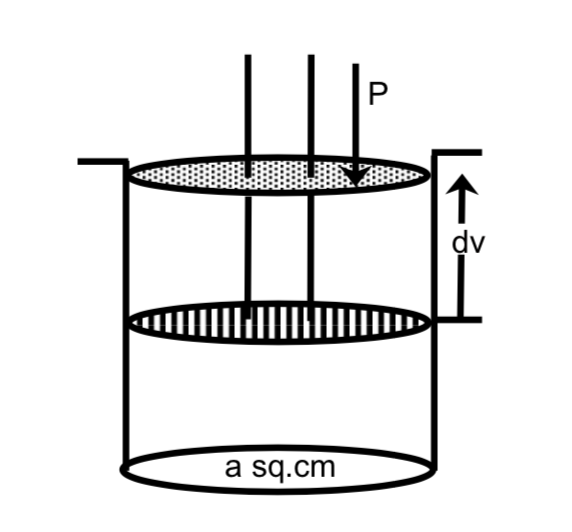b).Suppose n moles of a perfect gas is enclosed in a cylinder by a frictionless piston. The whole cylinder is kept in large constant temperature bath at T°K. Any change that would occur to the system would be isothermal.Suppose area of cross section of cylinder = a sq. cm

Work done in isothermal reversible expansion of an ideal gas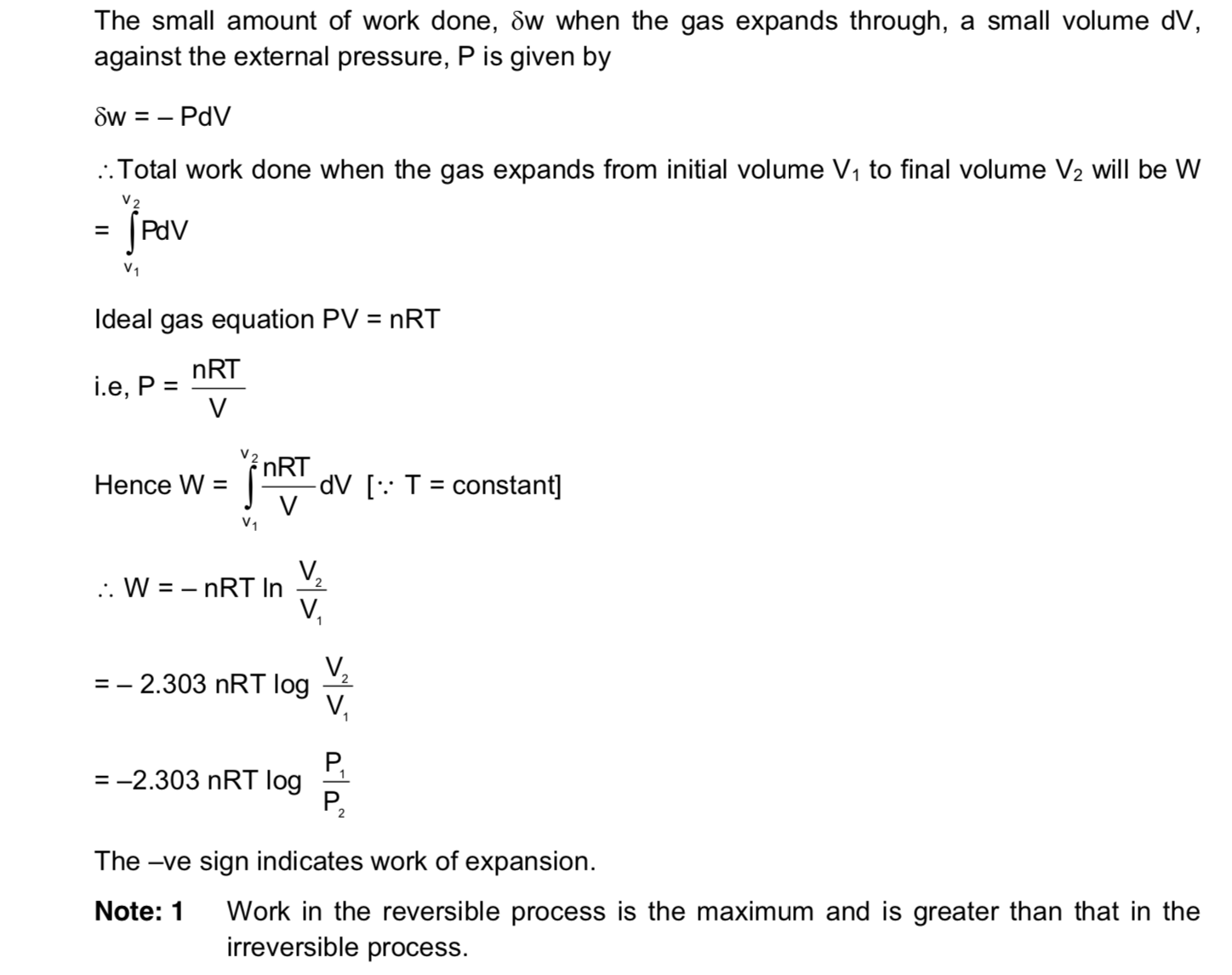Example :

70 gms of nitrogen gas was initially at 50 atm and 25°C
a) It was allowed to expand isothermally against a constant external pressure of one atmosphere. Calculate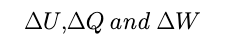,  assuming the gas to behave ideally.
b) Also find out the maximum work that would be obtained if the gas expanded reversibly and isothermally to one atmosphere.

Solution: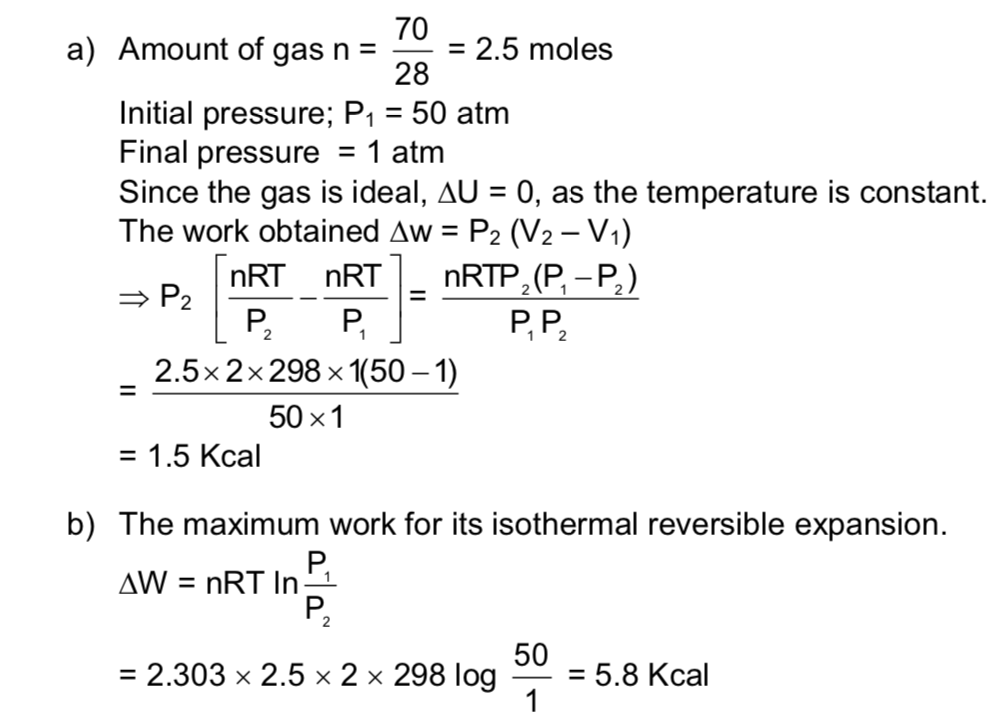Mole Concept - Concept of Limiting Reagent - Stoichiometric calculations

Stoichiometry deals with the calculation of the quantities of various reactants and products involved in a chemical reaction. The numerals used to balance a chemical equation are known as stoichiometric coefficients. These numerals are essential for solving problems based on chemical equation, hence called stoichiometric calculation.

In stoichiometric calculations, the mole relationship between different reactants and products are required as from them the mass-mass, mass-volume and volume-volume relationship between different reactants and products can be obtained.

Stoichiometric calculations can be carried out by two methods; Gravimetric Analysis and Volumetric Analysis. In the first method the amount of chemical species is determined by measurement of mass, whereas, in second method it is determined by measurement of volume.

Stoichiometric calculation can be carried in terms of two important concept:

(i) Mole Concept

(ii) Equivalent concept.

Mole Concept

A mole (symbol mol) is defined as the amount of substance that contains as many atoms, molecules, ions electrons or any other elementary entities as there are carbon atoms in exactly 12 gm of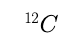. The number of atoms in 12 gm ofis called Avogadro’s number (N0).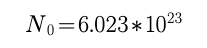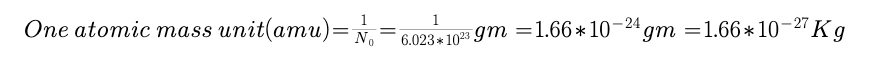The number of moles of a substance can be calculated by various means depending on data available, as follows.

Number of moles of molecules =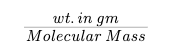Number of moles of atoms  =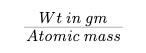Number of moles of gases =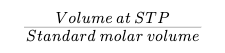Number of moles of particles e.g. atoms, molecules ions etc.  =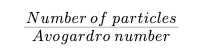Number of moles of solute = molarity *   volume of solution in litres =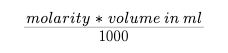for a compound AxBy, y moles of A = x moles of B

Mole fraction = fraction of the substance in the mixture expressed in terms of mol is called its mol fraction (X)

E.g. for a mixture of substance A & B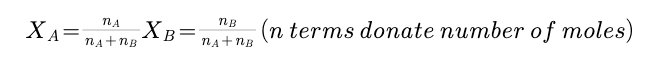Principle of Atom Conservation (POAC)

In chemical reaction atoms are conserved, so moles of atoms shall also be conserved. This is known as principle of atomic conservation. This principle is helpful in solving problems of nearly all stoichiometric calculations e.g.